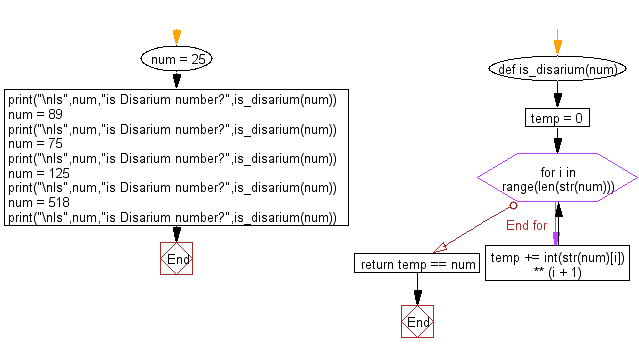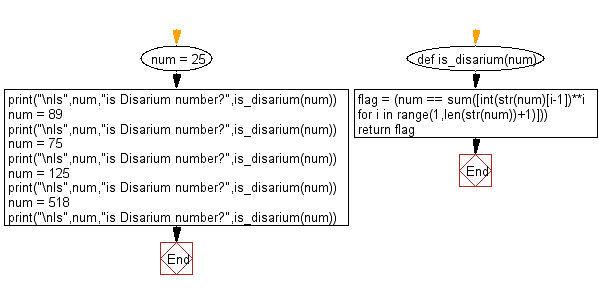﻿ Python Math: Check whether a given number is a Disarium number or unhappy number - w3resource# Python Math: Check whether a given number is a Disarium number or unhappy number

## Python Math: Exercise-88 with Solution

Write a Python program to check whether a given number is a Disarium number or unhappy number.

A Disarium number is a number defined by the following process:
Sum of its digits powered with their respective position is equal to the original number.
For example 175 is a Disarium number:
As 11+32+53 = 135
Some other DISARIUM are 89, 175, 518 etc.

Pictorial Presentation:Sample Solution-1:

Python Code:

``````def is_disarium(num):
temp = 0
for i in range(len(str(num))):
temp += int(str(num)[i]) ** (i + 1)
return temp == num

num = 25
print("\nIs",num,"is Disarium number?",is_disarium(num))
num = 89
print("\nIs",num,"is Disarium number?",is_disarium(num))
num = 75
print("\nIs",num,"is Disarium number?",is_disarium(num))
num = 125
print("\nIs",num,"is Disarium number?",is_disarium(num))
num = 518
print("\nIs",num,"is Disarium number?",is_disarium(num))
```
```

Sample Output:

```Is 25 is Disarium number? False

Is 89 is Disarium number? True

Is 75 is Disarium number? False

Is 125 is Disarium number? False

Is 518 is Disarium number? True
```

Flowchart:## Visualize Python code execution:

The following tool visualize what the computer is doing step-by-step as it executes the said program:

Sample Solution-2:

Python Code:

``````def is_disarium(num):
flag = (num == sum([int(str(num)[i-1])**i for i in range(1,len(str(num))+1)]))
return flag

num = 25
print("\nIs",num,"is Disarium number?",is_disarium(num))
num = 89
print("\nIs",num,"is Disarium number?",is_disarium(num))
num = 75
print("\nIs",num,"is Disarium number?",is_disarium(num))
num = 125
print("\nIs",num,"is Disarium number?",is_disarium(num))
num = 518
print("\nIs",num,"is Disarium number?",is_disarium(num))
```
```

Sample Output:

```Is 25 is Disarium number? False

Is 89 is Disarium number? True

Is 75 is Disarium number? False

Is 125 is Disarium number? False

Is 518 is Disarium number? True
```

Flowchart:## Visualize Python code execution:

The following tool visualize what the computer is doing step-by-step as it executes the said program:

Python Code Editor:

Have another way to solve this solution? Contribute your code (and comments) through Disqus.

What is the difficulty level of this exercise?

Test your Programming skills with w3resource's quiz.

﻿

```>>> students = [{'name': 'John', 'score': 98}, {'name': 'Mike', 'score': 94}, {'name': 'Jennifer', 'score': 99}]